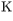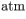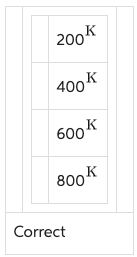# Problem: A tank is filled with an ideal gas at 400  and pressure of 1.00 .The tank is heated until the pressure of the gas in the tank doubles. What is the temperature of the gas?

###### FREE Expert Solution

Ideal gas equation:

$\overline{){\mathbf{P}}{\mathbf{V}}{\mathbf{=}}{\mathbf{n}}{\mathbf{R}}{\mathbf{T}}}$

T1 = 400K

P1 = 1atm

P2 = 2atm

100% (417 ratings)###### Problem Details

A tank is filled with an ideal gas at 400and pressure of 1.00.

The tank is heated until the pressure of the gas in the tank doubles. What is the temperature of the gas?